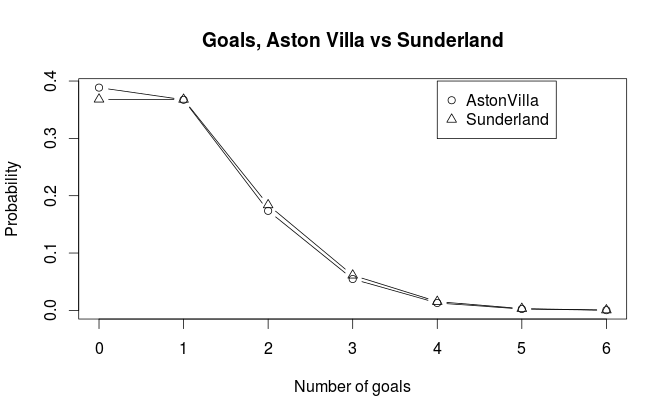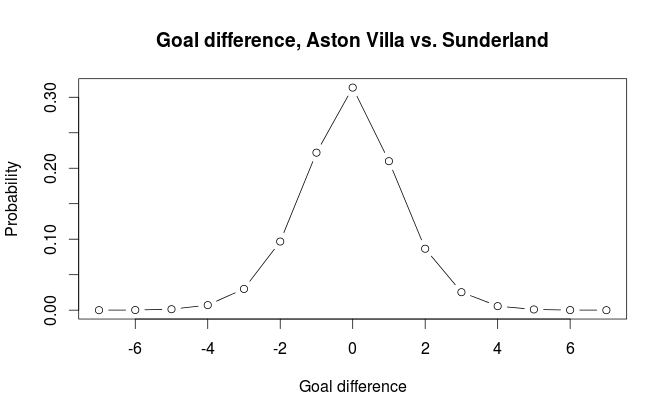# L-system in Python

The other day I was skimming trough articles on different subjects on Wikipedia. After a while I stumbled across the article on L-systems. In short, L-systems is method for generating fractals using simple string manipulations. You start out with an initial string, and for each character (called variable in L-system terminology) in that string you apply a set of rules that change that character into a set of new characters. Then you repeat n times. The resulting string is then a recipe for a graph or geometric structure. See the Wikipedia article for more details and examples.

I decided to try to implement this in Python. I only implemented the string manipulation engine and not a graphing tool. It was fairly easy, and the first method I came up with takes only about 15 lines of code and relies on recursion. A method without recursion is a couple of lines more, but probably more efficient. The user have to supply their own substitution rules by using a Python dictionary. Each key in the dictionary corresponds to a variable, and constants are defined implicitly by the production rules.

Here are two example from the Wikipedia article:

```
#Algae example
algaeRules = { "A" : "AB",
"B" : "A",}

algaeExample = lsystem("A", 7, algaeRules)
print(algaeExample)

#ABAABABAABAABABAABABAABAABABAABAAB
```
```#Sierpinski triangle example
sierpinskiRules = {"A": "B-A-B",
"B": "A+B+A"}

sierpinskiExample = lsystem("A", 3, sierpinskiRules)
print(sierpinskiExample)
#B-A-B+A+B+A+B-A-B-A+B+A-B-A-B-A+B+A-B-A-B+A+B+A+B-A-B
```

Here is the code for the lsystem() function, including the function that does not use recursion.

```def _applyRules(letter, rules):
"""Internal function for applying production rules on a single character."""
try:
return rules[letter]
except:
return letter

def lsystem(init, n, rulesDict):
"""Return the n-th iteration of a L-system (generated recursively)."""

if n <= 0:
return init
if n == 1:
return "".join([_applyRules(i, rulesDict) for i in init])
else:
newCond = "".join([_applyRules(i, rulesDict) for i in init])
return lsystem(newCond, n-1, rulesDict)

def lsystemNonRecursive(init, n, rulesDict):
"""Return the n-th iteration of a L-system."""

if n <= 0:
return init
else:
currentCond = init
while True:
currentCond = "".join([_applyRules(i, rulesDict) for i in currentCond])
n -= 1
if n == 0:
return currentCond
```

# Predicting football results with Poisson regression pt. 2

In part 1 I wrote about the basics of the Poisson regression model for predicting football results, and briefly mentioned how our data should look like. In this part I will look at how we can fit the model and calculate probabilities for the different match outcomes. I will also discuss some problems with the model, and hint at a few improvements.

Fitting the model with R
When we have the data in an appropriate format we can fit the model. R has a built in function glm() that can fit Poisson regression models. The code for loading the data, fitting the model and getting the summary is simple:

```#load data

#fit model and get a summary
model <- glm(Goals ~ Home + Team + Opponent, family=poisson(link=log), data=yrdta)
summary(model)
```

The summary function for fitting the model with data from Premier League 2011-2012 season gives us this (I have removed portions of it for space reasons):

(Edit September 2014: There was some errors in the estimates in the original version of this post. This was because I made some mistakes when I formated the data as described in part one. Thanks to Derek in the comments for pointing this out. )

```Coefficients:
Estimate Std. Error z value Pr(>|z|)
(Intercept)          0.45900    0.19029   2.412 0.015859 *
Home                 0.26801    0.06181   4.336 1.45e-05 ***
TeamAston Villa     -0.69103    0.20159  -3.428 0.000608 ***
TeamBlackburn       -0.40518    0.18568  -2.182 0.029094 *
TeamBolton          -0.44891    0.18810  -2.387 0.017003 *
TeamChelsea         -0.13312    0.17027  -0.782 0.434338
TeamEverton         -0.40202    0.18331  -2.193 0.028294 *
TeamFulham          -0.43216    0.18560  -2.328 0.019886 *
-----
OpponentSunderland  -0.09215    0.20558  -0.448 0.653968
OpponentSwansea      0.01026    0.20033   0.051 0.959135
OpponentTottenham   -0.18682    0.21199  -0.881 0.378161
OpponentWest Brom    0.03071    0.19939   0.154 0.877607
OpponentWigan        0.20406    0.19145   1.066 0.286476
OpponentWolves       0.48246    0.18088   2.667 0.007646 **
---
Signif. codes:  0 ‘***’ 0.001 ‘**’ 0.01 ‘*’ 0.05 ‘.’ 0.1 ‘ ’ 1
```

The Estimate column is the most interesting one. We see that the overall mean is e0.49 = 1.63 and that the home advantage is e0.26 = 1.30 (remember that we actually estimate the logarithm of the expectation, therefore we need to exponentiate the coefficients to get interpretable numbers). If we want to predict the results of a match between Aston Villa at home against Sunderland we could plug the estimates into our formula, or use the predict() function in R. We need to do this twice, one time to predict the number of goals Aston Villa is expected to score, and one time for Sunderland.

```#aston villa
predict(model, data.frame(Home=1, Team="Aston Villa", Opponent="Sunderland"), type="response")
# 0.9453705

#for sunderland. note that Home=0.
predict(model, data.frame(Home=0, Team="Sunderland", Opponent="Aston Villa"), type="response")
# 0.999
```

We see that Aston Villa is expected to score on average 0.945 goals, while Sunderland is expected to score on average 0.999 goals. We can plot the probabilities for the different number of goals against each other:We can see that Aston Villa has just a bit higher probability for scoring not goals than Sunderland. Sunderland has also just a tiny bit higher probablity for most other number of goals. Both teams have about the same probability of scoring exactly one goal. In general the pattern we see in the plot is consistent with what we would expect considering the expected number of goals.

Match result probabilities
Now that we have our expected number of goals for the two opponents in a match, we can calculate the probabilities for either home win (H), draw (D) and away win (A). But before we continue, there is an assumption in our model that needs to be discussed, namely the assumption that the goals scored by the two teams are independent. This may not be obvious since surely we have included information about who plays against who when we predict the number of goals for each team. But remember that each match is included twice in our data set, and the way the regression method works, each observation are assumed to be independent from the others. We’ll see later that this can cause some problems.

The most obvious way calculate the probabilities of the three outcomes is perhaps to look at goal differences. If we can calculate the probabilities for goal differences (home goals minus away goals) of exactly 0, less than 0, and greater than 0, we get the probabilities we are looking for. I will explain two ways of doing this, both yielding the same result (in theory at least): By using the Skellam distribution and by simulation.

Skellam distribution
The Skellam distribution is the probability distribution of the difference of two independent Poisson distributed variables, in other words, the probability distribution for the goal difference. R does not support it natively, but the VGAM package does. For our example the distribution looks like this:If we do the calculations we get the probabilities for home win, draw, away win to be 0.329, 0.314, 0.357 respectively.

```#Away
sum(dskellam(-100:-1, predictHome, predictAway)) #0.3574468
#Home
sum(dskellam(1:100, predictHome, predictAway)) #0.3289164
#Draw
sum(dskellam(0, predictHome, predictAway)) #0.3136368
```

Simulation
The second method we can use is simulation. We simulate a number of matches (10000 in our case) by having the computer draw random numbers from the two Poisson distributions and look at the differences. We get the probabilities for the different outcomes by calculating the proportion of different goal differences. The independence assumption makes this easy since we can simulate the number of goals for each team independently of each other.

``` set.seed(915706074)
nsim <- 10000
homeGoalsSim <- rpois(nsim, predictHome)
awayGoalsSim <- rpois(nsim, predictAway)
goalDiffSim <- homeGoalsSim - awayGoalsSim
#Home
sum(goalDiffSim > 0) / nsim #0.3275
#Draw
sum(goalDiffSim == 0) / nsim # 0.3197
#Away
sum(goalDiffSim < 0) / nsim #0.3528
```

The results differ a tiny bit from what we got from using the Skellam distribution. It is still accurate enough to not cause any big practical problems.

How good is the model at predicting match outcomes?
The Poisson regression model is not considered to be among the best models for predicting football results. It is especially poor at predicting draws. Even when the two teams are expected to score the same number of goals it rarely manages to assign the highest probability for a draw. In one attempt I used Premier League data from the last half of one season and the first half of the next season to predict the second half of that season (without refitting the model after each match day). It assigned highest probability to the right outcome in 50% of the matches, but never once predicted a draw.

Lets see at some other problems with the model and suggest some improvements.

One major problem I see with the model is that the predictor variables are categorical. This is a constraint that makes inefficient use of the available data since we get rather few data points per parameter (i.e per team). The model does for example not understand teams are more like each other than others and instead view each team in isolation. There has been some attempts at using Bayesian methods to incorporate information on which teams are better and which are poorer. Se for example this blog. If the teams instead could be reduced to numbers (by using some sort of rating system) we would get fewer parameters to estimate. We could then also incorporate an interaction term, something that is almost impossible with the categorical predictor variables we have. The interaction term in this case would be the effect of a team under or over estimating its opponent.

(As an aside, we could in fact interpret the coefficients in our model as a form of rating of a teams offensive and defensive strength)

Another way the model can be improved is to incorporate a time aspect. The most obvious way to do this is perhaps to weights to the matches such that more recent matches are more important than matches far back in time.

A further improvement would be to look at the level of different players, and not at a team as a whole. For example will a team with many injured players in a match most likely perform poorer than what you would expect. One could use weights to down weight the contribution of matches where this is a problem. A much more powerful idea would be to combine data on match lineup with a rating system for players. This could be used to infer a rating for the whole team in a specific match. In addition to correct for injured players it would also account for new players on a team and players leaving a team. The biggest problem with this approach is lack of available data in a format that is easy to handle.

I don’t think any of the improvements I have discussed here will solve the problem of predicting draws since it originates in the independent Poisson assumption, although I think they could improve predictions in general. To counter the problem of predicting draws I think a very different model would have to be used. I would also like to mention that the improvements I have suggested here are rather general, and could be incorporated in many other prediction models.